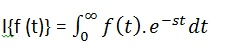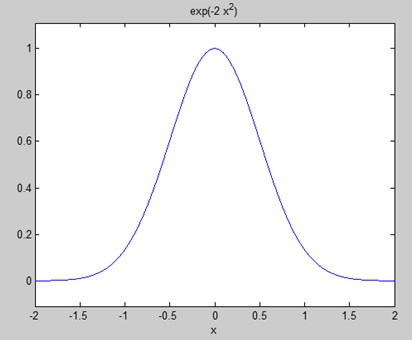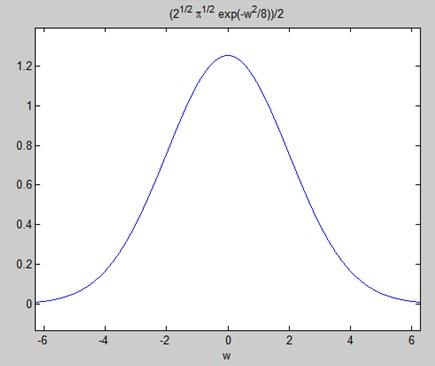# MATLAB - Transforms

#### Data Preprocessing for Machine Learning using MATLAB

30 Lectures 4 hours

#### Image Processing Toolbox in MATLAB

17 Lectures 3 hours

#### Matlab - The Complete Course

Best Seller

37 Lectures 5 hours

MATLAB provides command for working with transforms, such as the Laplace and Fourier transforms. Transforms are used in science and engineering as a tool for simplifying analysis and look at data from another angle.

For example, the Fourier transform allows us to convert a signal represented as a function of time to a function of frequency. Laplace transform allows us to convert a differential equation to an algebraic equation.

MATLAB provides the laplace, fourier and fft commands to work with Laplace, Fourier and Fast Fourier transforms.

## The Laplace Transform

The Laplace transform of a function of time f(t) is given by the following integral −Laplace transform is also denoted as transform of f(t) to F(s). You can see this transform or integration process converts f(t), a function of the symbolic variable t, into another function F(s), with another variable s.

Laplace transform turns differential equations into algebraic ones. To compute a Laplace transform of a function f(t), write −

```laplace(f(t))
```

### Example

In this example, we will compute the Laplace transform of some commonly used functions.

Create a script file and type the following code −

```syms s t a b w

laplace(a)
laplace(t^2)
laplace(t^9)
laplace(exp(-b*t))
laplace(sin(w*t))
laplace(cos(w*t))
```

When you run the file, it displays the following result −

```ans =
1/s^2

ans =
2/s^3

ans =
362880/s^10

ans =
1/(b + s)

ans =
w/(s^2 + w^2)

ans =
s/(s^2 + w^2)
```

## The Inverse Laplace Transform

MATLAB allows us to compute the inverse Laplace transform using the command ilaplace.

For example,

```ilaplace(1/s^3)
```

MATLAB will execute the above statement and display the result −

```ans =
t^2/2
```

### Example

Create a script file and type the following code −

```syms s t a b w

ilaplace(1/s^7)
ilaplace(2/(w+s))
ilaplace(s/(s^2+4))
ilaplace(exp(-b*t))
ilaplace(w/(s^2 + w^2))
ilaplace(s/(s^2 + w^2))
```

When you run the file, it displays the following result −

```ans =
t^6/720

ans =
2*exp(-t*w)

ans =
cos(2*t)

ans =
ilaplace(exp(-b*t), t, x)

ans =
sin(t*w)

ans =
cos(t*w)
```

## The Fourier Transforms

Fourier transforms commonly transforms a mathematical function of time, f(t), into a new function, sometimes denoted by or F, whose argument is frequency with units of cycles/s (hertz) or radians per second. The new function is then known as the Fourier transform and/or the frequency spectrum of the function f.

### Example

Create a script file and type the following code in it −

```syms x
f = exp(-2*x^2);     %our function
ezplot(f,[-2,2])     % plot of our function
FT = fourier(f)      % Fourier transform
```

When you run the file, MATLAB plots the following graph −The following result is displayed −

```FT =
(2^(1/2)*pi^(1/2)*exp(-w^2/8))/2
```

Plotting the Fourier transform as −

```ezplot(FT)
```

Gives the following graph −## Inverse Fourier Transforms

MATLAB provides the ifourier command for computing the inverse Fourier transform of a function. For example,

```f = ifourier(-2*exp(-abs(w)))
```

MATLAB will execute the above statement and display the result −

```f =
-2/(pi*(x^2 + 1))
```